Create Custom column values from the dataset

(This is like creating a UDHC value at the report or analysis level. This can be any real life scenario where we can add calculated columns and values as a new dimension value from the dataset itself.)

Scenario:

The base finance data has Transaction date wise nature of ledgers, ledgers and their amount.

Requirement:

Create profit (net amount) i.e. income – expense. The income-expense category comes under Nature of Ledger column. Profit (net amount) will be created as another dimension value under this Nature of Ledger.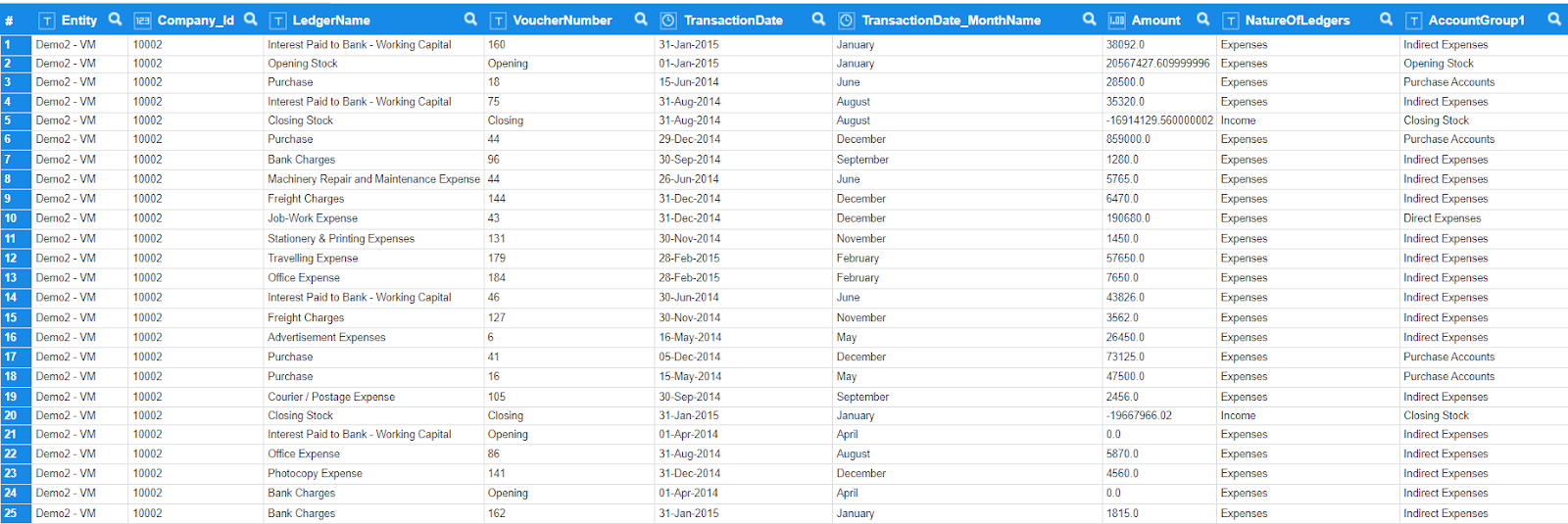As shown in the above crosstab, we have nature of ledgers (Income and Expense) and account group wise amount for various months. In this scenario, we will be deriving the Net profit amount (i.e. Income –Expense) from the dataset and it will be created under Nature of Ledgers.

Solution:

The requirement can be achieved in following easy steps. As mentioned above, the finance data has Transaction date wise nature of ledgers, account group and their amount.

1. Create copy of existing dataset - Need to create a dataset as a replica of the current one or take a copy of the current one for ease of use.

2. Filter ‘Nature of ledger’ column - Since the calculation is Net Profit Amount (i.e. Income – Expense), rest of the nature of ledger values such as assets, liabilities, etc. are not required in the dataset. Hence we will filter this for only Income and Expense transactions.

 Nature of Ledgers Account Group Amount Expenses Direct Expenses 2000 Expenses Indirect Expenses 3000 Income Closing Stock 1000 Income Sales accounts 5000

3. Create custom column based on ‘Nature of ledger’ column values - To calculate the Net amount from Income and expense we will need to create the 'Income amount’ and ‘Expense amount’ in two new columns i.e. two custom columns. Income amount will be for the type 'Income' ledger and Expense amount will be for the type 'Expense'.

 Nature of Ledgers Account Group Amount Income Amount Expense Amount Expenses Direct Expenses 2000 0 2000 Expenses Indirect Expenses 3000 0 3000 Income Closing Stock 1000 1000 0 Income Sales accounts 5000 5000 0

4. Remove unwanted columns and aggregate- Remove all the unwanted columns such as Nature of Ledgers (as we have separated the amount columns now), account group and amount and aggregate the dataset.

 Income Amount Expense Amount 6000 5000

5. Create custom column for ‘Net amount’ - Create another column for calculating the ‘Net Amount’ - having formula Income Amount -Expense Amount.

 Income Amount Expense Amount Net Amount 6000 5000 1000

6. Append new dataset with original dataset - Now get back to the Parent dataset where we have all the columns (Nature of Ledgers, Account Group, Date, Voucher no., Amount, etc.). APPEND the child dataset (which is ready by step 5) with the main raw data.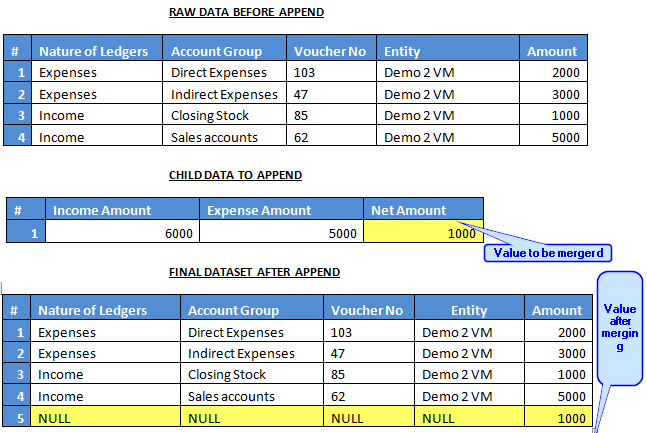7. Add ‘Net Profit’ column value to ‘Nature of ledger’ - After UNION, NULL values will be filled under the Nature of Ledger column. Replace the NULL values of Nature of Ledger column as “Net Amount” text and make the final dataset ready.

 Nature of Ledgers Account Group Amount Expenses Direct Expenses 2000 Expenses Indirect Expenses 3000 Income Closing Stock 1000 Income Sales accounts 5000 Net Amount NULL 1000

For detailed steps in achieving in Smarten, please refer to the below content.

Step 1: Create copy of existing dataset

To begin with, we need to either create a new dataset as a replica of the current one OR get a copy of the current dataset. This is for creating the child dataset with aggregation, filter, custom columns, etc. Keep only the required columns that you need in the analytical level in the child dataset before aggregation.

Steps to copy dataset:-

1. Mark the Dataset and make a copy of the dataset.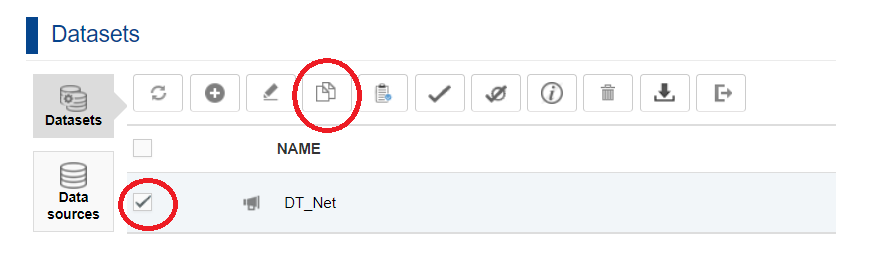2. Now give the name to the copied dataset and click on OK.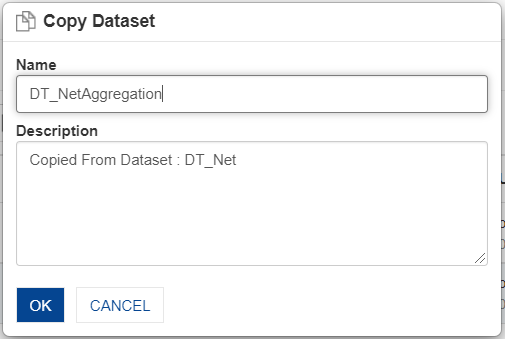Below is the dataset to be aggregated with the required columns (Transaction date month name, Amount, Nature of Ledgers, and Account Group 1).Step 2: Filter ‘Nature of ledger’ column

Let us consider creating the Net amount value from the ledgers (Income and Expense) now. Hence we will keep only Income and Expense under the Nature of Ledgers column of the aggregated dataset. You can filter them out as per the below steps.

Right click on Nature of Ledger column -> Filter -> Custom ->Nature of Ledger= Income OR Nature of Ledger= Expenses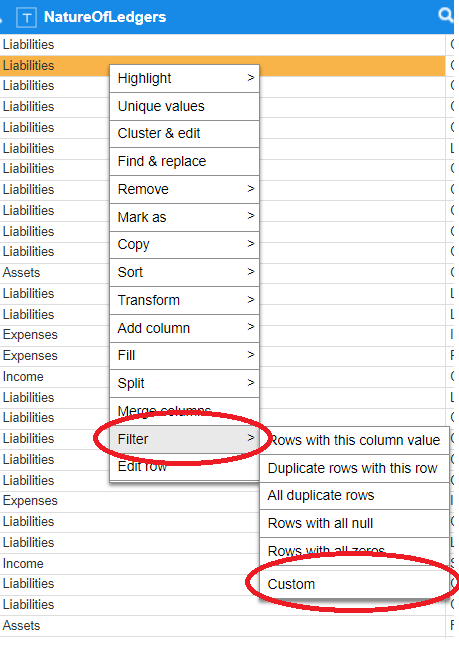Below is the resultant dataset after filtering out the required Nature of Ledgers (i.e. Income and expenses).Step 3: Create custom column based on ‘Nature of ledger’ column values

To calculate the Net amount from Income and expense we will need to bring the income amount and expense amount in new columns i.e. two custom columns.

Right click (on any column) -> Add column -> Custom

Give the expression as:-

IncomeAmount = ifCase(NatureOfLedgers == “Income” , Amount , 0) and

ExpensesAmount = ifCase(NatureOfLedgers == “Expenses” , Amount , 0).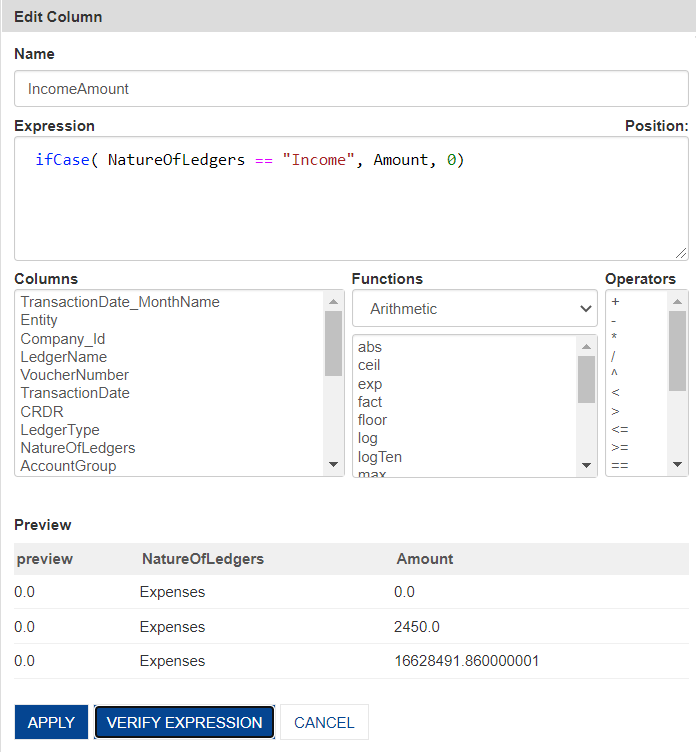Two new columns (ExpensesAmount and IncomeAmount) will be created as shown below.Step 4: Remove unwanted columns and aggregate

Since we have separated the amount for Income and expense, we do not require the ‘NatureOfLedgers’ column here in this dataset. So remove the ‘NatureOfLedgers’column and aggregate the dataset with other columns.

To aggregate, click on the Aggregate option on the menu bar at the top as shown in the figure below.Below is the resultant dataset after aggregating the data. The resultant dataset will have the required columns and the aggregated amount of income and expense as below.Step 5: Create custom column for ‘Net amount’

Now, subtract these two ledger values to calculate the Net Profit Amount.

Right click (on any column) -> Add column -> Custom – NetAmount

Given the expression as: - IncomeAmount - ExpenseAmount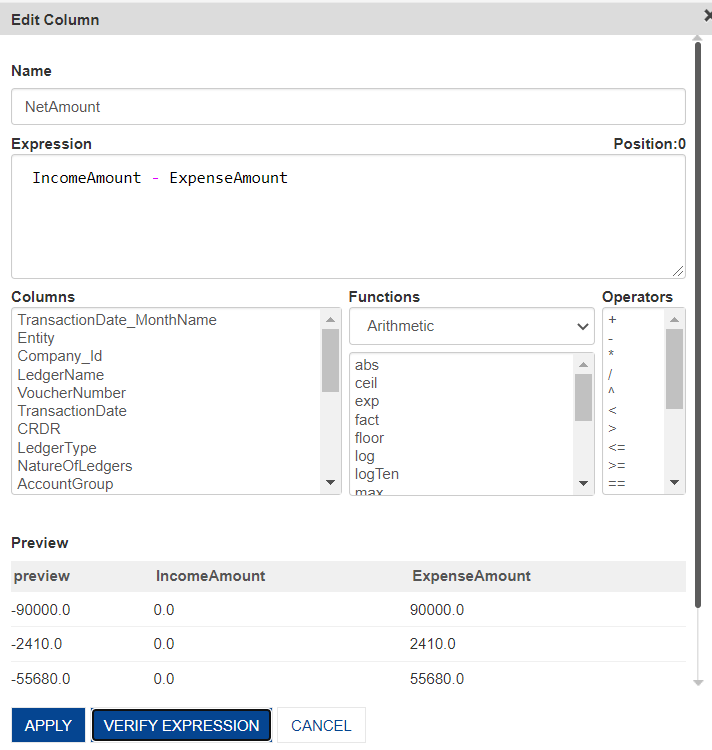Below is the dataset after calculating the Net Amount and ready to append with the main dataset.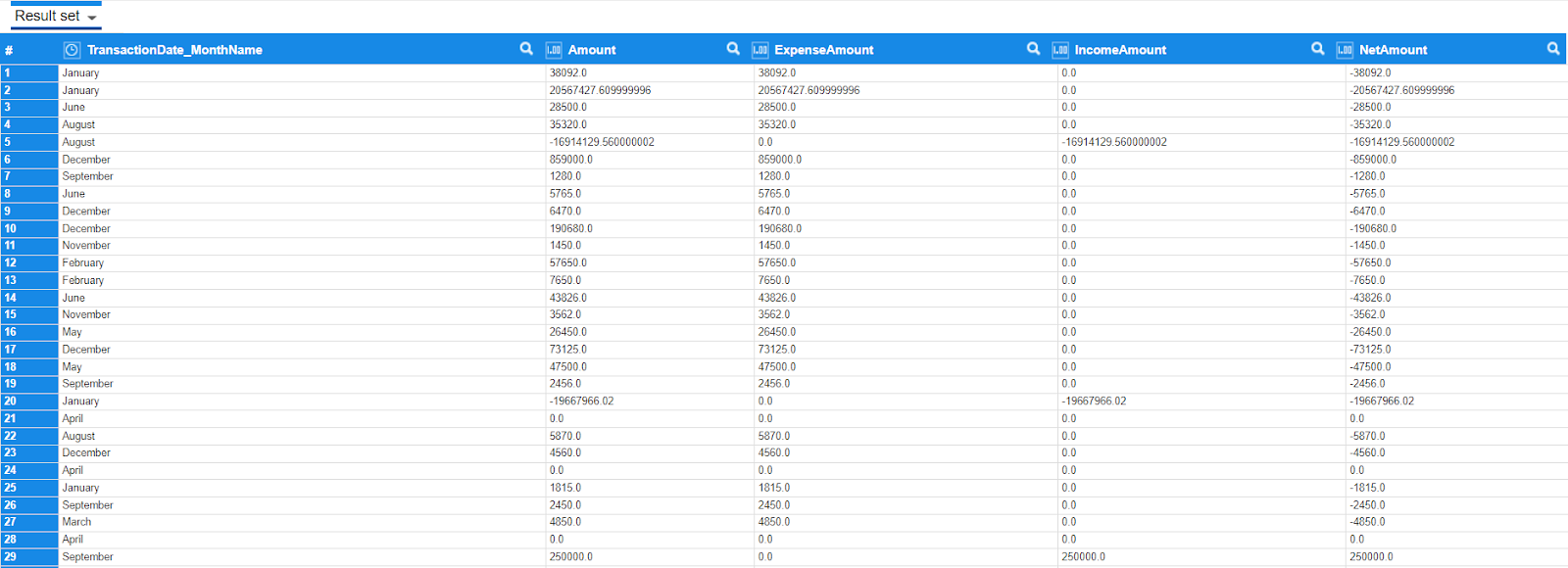Step 6: Append new dataset with original dataset

Now bring the aggregated dataset (of Step 5) in the main dataset and append the same (Blend-Append). To append the dataset, click on the Blend-Append button on the toolbar.Then map the columns of both the datasets as below. It is important to map the Amount (In the main dataset) with the Net Amount (in the aggregated dataset) apart from the other column mapping as Net Profit Amount value will be considered under Gross sales column.

1. TransactionDate_Monthname = TransactionDate_Monthname

2. Amount = NetAmountStep 7: Add ‘Net Profit’ column value to ‘Nature of ledger’

Null values will be inserted in the Nature of Ledgers dimension column as there were no values from the aggregated dataset. So Null represents our Net Amount values. We need to replace the Null with the name “Net Amount” here. So the values in the Nature of Ledgers column would be Income, Expense and Net Amount.

Right click (on Nature of Ledgers column) -> Unique Values -> Change Null to Net Amount.

Result:

Create a crosstab with the below columns in the respective sections.

• Transaction_Monthname in column

• NatureOfLedger and AccountGroup1 in Row

• Amount in Data

• NatureOfLedgers in Filter (Select Income, Expenses and NetAmount)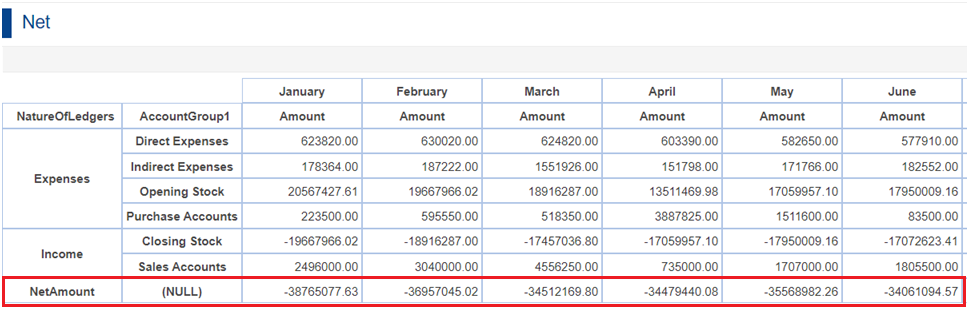NatureOfLedgers has 3 values now - Expenses, Income and NetAmount as shown in the above crosstab.